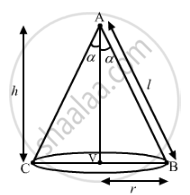# Show that the semi-vertical angle of the cone of the maximum volume and of given slant height is cos^(-1)(1/sqrt3) - Mathematics

Show that the semi-vertical angle of the cone of the maximum volume and of given slant height is cos^(-1)(1/sqrt3)

#### Solution

Let us consider the following variables:
h = height of the cone
l = slant height of the cone (given)
r = radius of the base of the cone
α = semi vertical angle of the coneLet us assume that V' is the volume of the cone which has to be maximised.

We know that

V' = 1/3πr^2h                           ...(1)

From the figure, we have:

l^2=r^2+h^2⇒l^2−h^2=r^2

On substituting the value of r2 in equation (1), we get:

V' = 1/3π(l^2−h^2)h=1/3π(l^2h−h^3)

On differentiating with respect to h, we get:

dV'/dh=1/3π(l^2−3h^2)                  ...(2)

For maximum volume of V, let us put (dV')/(dh)=0.
So, from equation (2), we have:

1/3π(l^2−3h^2)=0⇒h=l/sqrt3      (∵ h,l >0)

Again, differentiating equation (2) with respect to h, we get:

(d^2V'/dh^2)=1/3π(−6h)=−2πh

∴ ((d^2V')/(dh^2))_(h=l/sqrt3)=−2πl/sqrt3<0

Thus, the volume of the cone is maximum at

h=l/sqrt3

From the figure, we have:

cosα =h/l⇒cosα =l/lsqrt3=1/sqrt3

⇒α=cos^(−1)(1/sqrt3)

∴ The semi-vertical angle is

cos^(−1)(1/sqrt3).

Concept: Simple Problems on Applications of Derivatives
Is there an error in this question or solution?
2013-2014 (March) Delhi Set 1

Share